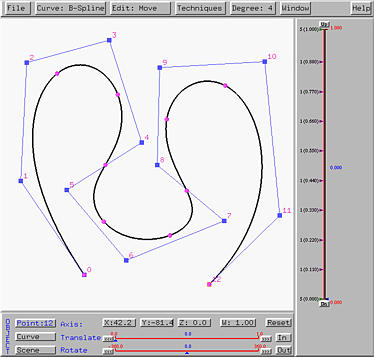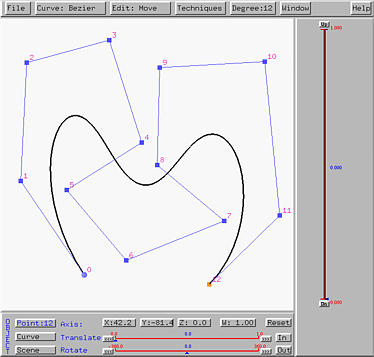# Creating CurvesTo create a curve in the xy-plane, you need the following steps:

1. Select Edit, followed by Create. This will put the curve system in the insert and the cursor is changed to a cross. Note that the menu item shows Edit: Create, meaning that the system is in the insert mode.

2. The second step is to specify a curve type. This system supports four types of curves: Bézier, rational Bézier, B-spline and NURBS curves. All four types require a set of control points.

Select Curve, followed by New Curve Segment, followed by the curve type you want. For example, if you choose the curve type to be B-Spline, then the menu item shows Curve: B-Spline.

3. For Bézier and rational Bézier curves, the degree of the curve is the number of control points minus 1. For example, if a Bézier curve is defined by nine control points, its degree is 8. For B-spline and NURBS curves, one need to specify the degree of the desired curve. The default degree for a B-spline and NURBS curve is 3. But, you can change this value by selecting Degree, followed by the desired degree. This system supports degree up to 10 for B-spline and NURBS curves, which is sufficient for more design purposes. If we choose 4, the menu item will show Degree: 4

4. Now, you can move the cross pointer into the drawing canvas and click to put control points there. In the figure below, we put 13 control points on the drawing canvas. Please note that the control point IDs start with 0. As a result, the control points are numbered from 0 to 12.

5. Finally, we can display the curve by selecting Curve, followed by Show Curve Segment. We have three choices: With Uniform Knots, With Clamped Knots, and With Closed Knots. Meanwhile, let us select With Clamped Knots, since our study centers around this type of curves. Then, we shall see the following B-spline curve of degree 4, with clamped knots, on the drawing canvas.6. Recall that the right hand side of the vertical slider is for curve tracing and the left hand side shows the knots. Each knot is shown in a form of m (d.ddd), where m and d.ddd are the multiplicity and value of a knot, respectively. After a curve is generated, all knots are equally spaced.

As mentioned earlier, Bézier and rational Bézier curves do not require a degree. One can just put control points on the drawing canvas. As you are clicking, the curve will be generated on-the-fly. So, creating Bézier and rational Bézier curves are simpler than creating B-spline and NURBS curves. The following figure shows a Bézier curve generated from the same set of control points that were used to created the above B-spline curve. Note that since there are 13 control points, this Bézier curve should have degree 12 as shown in the Degree: 12.If you compare this Bézier curve with the above B-spline curve, you will see that the B-spline curve of degree 4 follows the control polygon more closely than the Bézier curve of degree 12 does. This is a good reason for using B-spline and NURBS curves, because it allows to use a lower degree curve to follow the given control polyline closely. However, Bézier curves are simpler and easier to understand and implement.